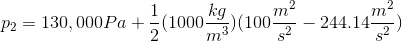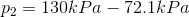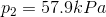# AP Physics B : Fluid and Gas Mechanics

## Example Questions

### Example Question #1 : Understanding Bernoulli's Equation

An incompressible fluid flows without viscosity at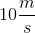through a pipe of radius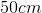. Without changing height, it flows into a pipe whose radius is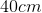. If the water pressure is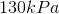in the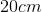diameter pipe, what is its pressure in the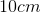diameter pipe?

The mass density of the fluid is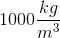.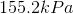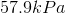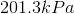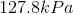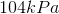Explanation:

If the fluid is incompressible and flows without viscosity in a confined space, then an equal volume must move past any point in that space in the same time interval, even if the geometry of the confines changes.  An equal volume must pass Pointsandin the same time in the figure.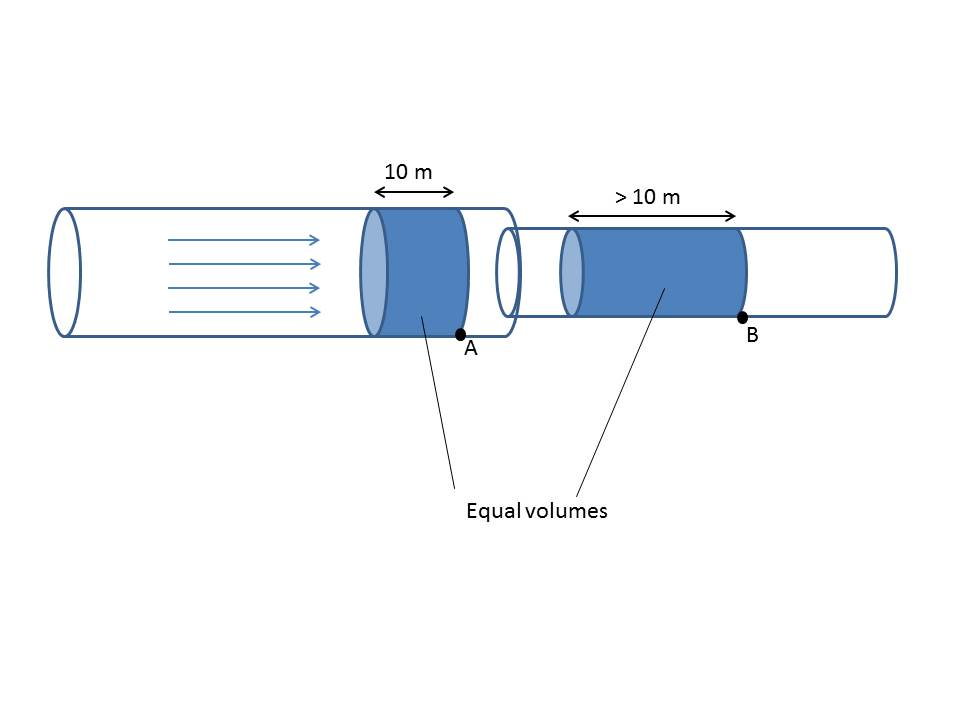Since the diameter of the section on the right is smaller, the fluid must move faster in that region for an equal volume to pass by pointand pointin the same time.

In this problem, the flow speed in the larger section of pipe is, so a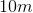cylinder of fluid passes pointin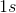. What length cylinder passes pointin that same second? It must be an equal volume, so we can use the equation for volume of a cylinder, where height is equal to the length of the pipe.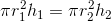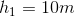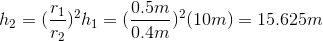Hence, the flow speed in the smaller section of pipe is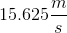, since this is the distance in the pipe that the fluid must travel per second.

Now use Bernoulli’s principle, which expresses conservation of energy in fluid flow as:

Pressure + kinetic energy of flow + fluid potential energy = constant

Fluid potential energy depends on height (like gravitational potential energy), and so does not change from one part of the pipe to the other. So, for this case, Bernoulli's principle becomes: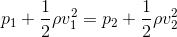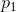is the pressure in the larger pipe,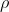is the mass density of the fluid (constant for incompressible fluid), and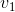and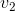are, respectively, the flow speeds in the larger and smaller sections of the pipe. Putting in the known values and solving for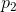yields: